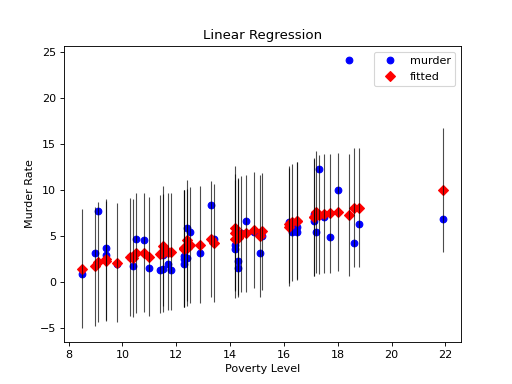# statsmodels.graphics.regressionplots.plot_fit¶

statsmodels.graphics.regressionplots.plot_fit(results, exog_idx, y_true=None, ax=None, vlines=True, **kwargs)[source]

Plot fit against one regressor.

This creates one graph with the scatterplot of observed values compared to fitted values.

Parameters
resultsResults

A result instance with resid, model.endog and model.exog as attributes.

exog_idx

Name or index of regressor in exog matrix.

y_truearray_like. optional

If this is not None, then the array is added to the plot.

axAxesSubplot, optional

If given, this subplot is used to plot in instead of a new figure being created.

vlinesbool, optional

If this not True, then the uncertainty of the fit is not plotted.

**kwargs

The keyword arguments are passed to the plot command for the fitted values points.

Returns
Figure

If ax is None, the created figure. Otherwise the figure to which ax is connected.

Examples

Load the Statewide Crime data set and perform linear regression with poverty and hs_grad as variables and murder as the response

>>> import statsmodels.api as sm
>>> import matplotlib.pyplot as plt

>>> data = sm.datasets.statecrime.load_pandas().data
>>> murder = data['murder']

>>> X["constant"] = 1
>>> y = murder
>>> model = sm.OLS(y, X)
>>> results = model.fit()


Create a plot just for the variable ‘Poverty’:

>>> fig, ax = plt.subplots()
>>> fig = sm.graphics.plot_fit(results, 0, ax=ax)
>>> ax.set_ylabel("Murder Rate")
>>> ax.set_xlabel("Poverty Level")
>>> ax.set_title("Linear Regression")

>>> plt.show()